﻿ 一种变阻尼稳定伞的气动分析 Aerodynamic Analysis of a Variable Damping Stabilized Parachute

Modeling and Simulation
Vol. 08  No. 01 ( 2019 ), Article ID: 28896 , 7 pages
10.12677/MOS.2019.81005

Aerodynamic Analysis of a Variable Damping Stabilized Parachute

Kuan Zhang, Xiaoning Fu

School of Electromechanical Engineering, Xidian University, Xi’an Shaanxi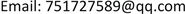Received: Jan. 26th, 2019; accepted: Feb. 10th, 2019; published: Feb. 18th, 2019ABSTRACT

The hose-paradrogue is prone to swing motion due to the interference of airflow during air refueling, which makes the docking process more difficult. The flexible hose-paradrogue system in flexible aerial refueling is simulated and analyzed by Computational Fluid Dynamics simulation technology, which provides reference for the design of flexible hose-cone sleeve controller and improves the controllability of flexible hose-cone sleeve system.

Keywords:Variable Damping Stabilization Umbrella, Computational Fluid Dynamics Simulation, ControllabilityCopyright © 2019 by authors and Hans Publishers Inc.

This work is licensed under the Creative Commons Attribution International License (CC BY).

http://creativecommons.org/licenses/by/4.0/1. 引言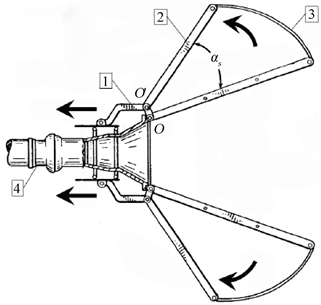Figure 1. Structural sketch of a variable damping stabilization umbrella

2. 变阻尼稳定伞设计及伞面形状的确定

3. CFD数值模拟

3.1. 稳定伞模型

3.2. 仿真结果分析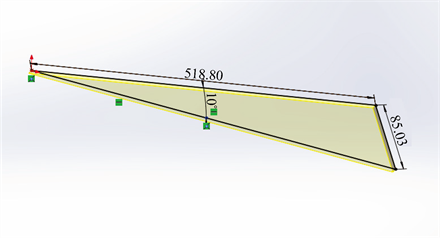(a)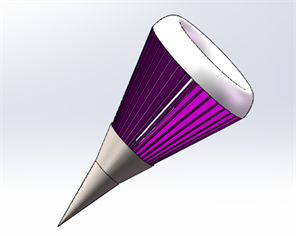(b)

Figure 2. (a) 10-degree umbrella support size; (b) SolidWorks model of paradrogue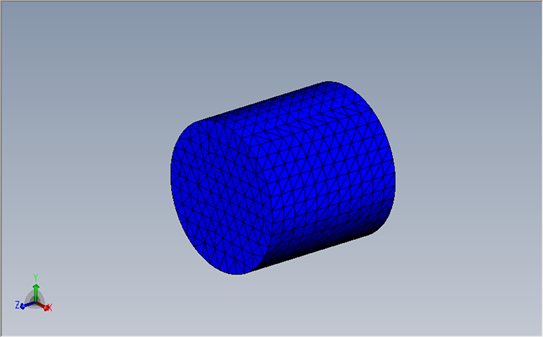Figure 3. The computational domain of steady parachute flow field(a) αs = 10˚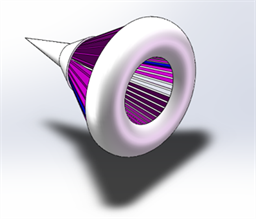(b) αs = 20˚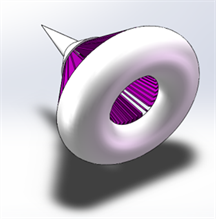(c) αs = 30˚

Figure 4. The shape of three conical sleeve stabilized parachutes

$\text{T}={\text{T}}_{\text{a}}-0.0065H$ (1)

$\frac{\text{p}}{{\text{P}}_{\text{a}}}={\left(\frac{\text{T}}{{\text{T}}_{a}}\right)}^{5.25588}$ (2)

$\frac{\rho }{{\rho }_{\text{a}}}={\left(\frac{\text{T}}{{\text{T}}_{a}}\right)}^{4.25588}$ (3)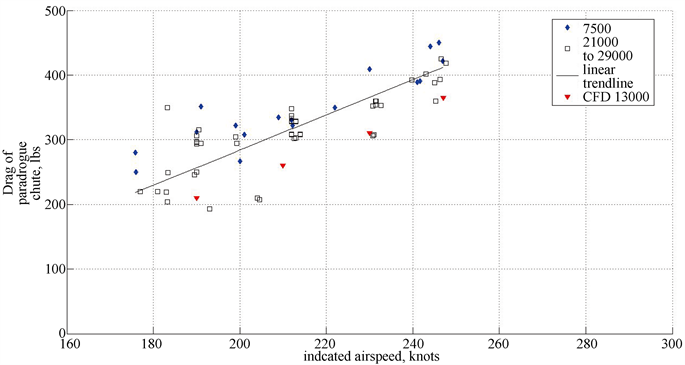Figure 5. Comparison of CFD simulation results with flight test data

4. 模拟结果与分析

$\text{D=}\frac{1}{2}{\rho }_{\infty }{\text{V}}_{\infty }{}^{2}{\text{S}}_{\text{ref}}\text{Cd}$ (4)

$\text{Cd=}\mathrm{f}\left({\text{V}}_{\infty },{\alpha }_{s}\right)$ (5)

$\text{Cd}\approx \text{f}\left({\alpha }_{\text{s}}\right)$ (6)

$\text{Cd}\approx \text{A}{\alpha }_{\text{s}}{}^{2}+B{\alpha }_{\text{s}}+C$ (7)

$\text{Cd}\approx 0.0003{\alpha }_{\text{s}}{}^{2}-\text{0}\text{.003}{\alpha }_{\text{s}}+0.65$ (8)(a) ${\alpha }_{s}$ = 10˚(b) ${\alpha }_{s}$ =20˚(c) ${\alpha }_{s}$ = 10˚

Figure 6. Ma = 0.5, streamline diagram of leeward section of stable umbrella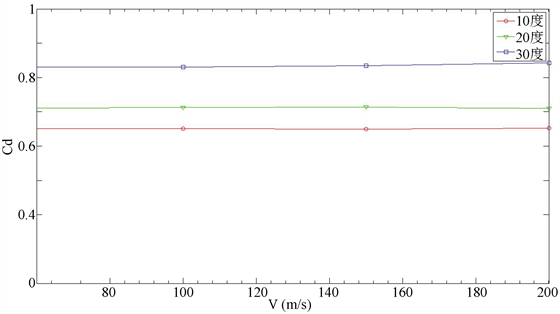Figure 7. Resistance coefficient-velocity curve of stable parachute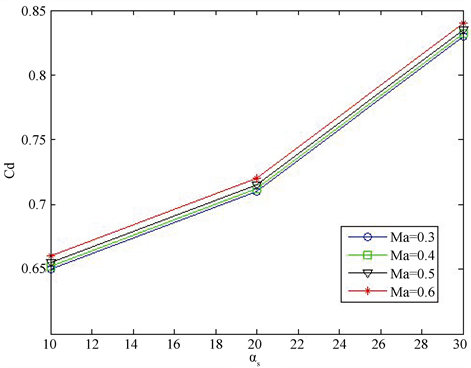Figure 8. The relationship curve between drag coefficient and brace angle of stabilized parachute

5. 总结

Aerodynamic Analysis of a Variable Damping Stabilized Parachute[J]. 建模与仿真, 2019, 08(01): 31-37. https://doi.org/10.12677/MOS.2019.81005

1. 1. Ro, K. and Basaran, E. (2006) Aerodynamic Investigations of Paradrogue Assembly in Aerial Refueling System. AIAA Aerospace Sciences Meeting & Exhibit, 68-70.

2. 2. 王海涛, 董新民, 窦和锋, 等. 软管锥套式空中加油系统建模与特性分析[J]. 北京航空航天大学学报, 2014, 40(1): 92-98.

3. 3. 张进, 袁锁中, 龚全铨. 空中加油软管-锥套动态建模及其飘摆运动抑制[J]. 科学技术与工程, 2015, 15(8): 134-139.

4. 4. Williamson, W.R., Reed, E., Glenn, G.J., et al. (2010) Controllable Drogue for Automated Aerial Refueling. Journal of Aircraft, 47, 515-527. https://doi.org/10.2514/1.44758

5. 5. Kirkland, W.L. (2010) Variable Speed Drogue US2011/0108669.

6. 6. Ro, K., Ahmad, H. and Kamman, J. (2009) Dynamic Modeling and Simulation of Hose-Paradrogue Assembly for Mid-Air Operations. AIAA Journal, Seattle, Washington, 6-9 April 2009, 1-14.

7. 7. 吴成林. 空中加油软管—锥套系统动态特性研究[D]: [硕士学位论文]. 南京: 南京航空航天大学, 2015.

8. 8. 吴玲, 孙永荣, 黄斌, 等. 不确定环境下的软管-锥套建模及控制研究[J]. 计算机工程与应用, 2017(18): 250-256.

9. 9. Ro, K. and Kamman, J.W. (2010) Modeling and Simulation of Hose-Paradrogue Aerial Refueling Systems. Journal of Guidance, Control, and Dynamics, 33, 53-63. https://doi.org/10.2514/1.45482

10. 10. 张进, 袁锁中, 龚全铨, 等. 空中加油软管-锥套空中飘摆运动建模与控制[J]. 系统仿真学报, 2016, 28(2): 388-395.